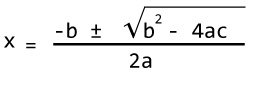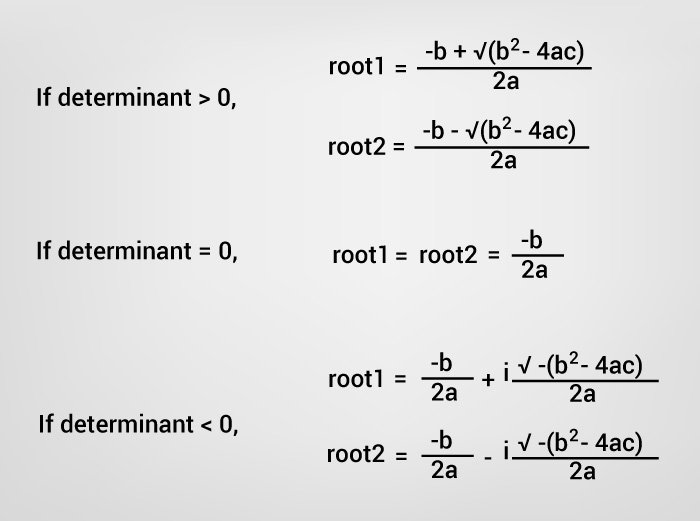# C++ Program to Find All Roots of a Quadratic Equation

This program accepts coefficients of a quadratic equation from the user and displays the roots (both real and complex roots depending upon the discriminant).

To understand this example, you should have the knowledge of following C++ programming topics:

For a quadratic equation ax2+bx+c = 0 (where a, b and c are coefficients), it's roots is given by following the formula.The term `b2-4ac` is known as the discriminant of a quadratic equation. The discriminant tells the nature of the roots.

• If discriminant is greater than 0, the roots are real and different.
• If discriminant is equal to 0, the roots are real and equal.
• If discriminant is less than 0, the roots are complex and different.## Example: Roots of a Quadratic Equation

``````#include <iostream>
#include <cmath>
using namespace std;

int main() {

float a, b, c, x1, x2, discriminant, realPart, imaginaryPart;
cout << "Enter coefficients a, b and c: ";
cin >> a >> b >> c;
discriminant = b*b - 4*a*c;

if (discriminant > 0) {
x1 = (-b + sqrt(discriminant)) / (2*a);
x2 = (-b - sqrt(discriminant)) / (2*a);
cout << "Roots are real and different." << endl;
cout << "x1 = " << x1 << endl;
cout << "x2 = " << x2 << endl;
}

else if (discriminant == 0) {
cout << "Roots are real and same." << endl;
x1 = (-b + sqrt(discriminant)) / (2*a);
cout << "x1 = x2 =" << x1 << endl;
}

else {
realPart = -b/(2*a);
imaginaryPart =sqrt(-discriminant)/(2*a);
cout << "Roots are complex and different."  << endl;
cout << "x1 = " << realPart << "+" << imaginaryPart << "i" << endl;
cout << "x2 = " << realPart << "-" << imaginaryPart << "i" << endl;
}

return 0;
}``````

Output

```Enter coefficients a, b and c: 4
5
1
Roots are real and different.
x1 = -0.25
x2 = -1
```

In this program, `sqrt()` library function is used to find the square root of a number.